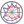Course title
Fundamentals of Electric Circuits
 horie ryotaLEE HEEYOUNG
Course description
Electrical circuit theory is a required subject in the field of electrical engineering and applicable to many disciplines. In this course, students learn circuit theory with a focus on Ohm’s law and Kirchhoff’s law and acquire principle theory required for analysis of alternating current (AC) circuits. Then, learning contents are progressed from basic circuits to networks. Students learn array expressing features of a network.
Purpose of class
The purpose of this course is to learn principle concepts required in electrical circuit, principal theory required for analysis of AC circuits, and concepts and mathematical expressions required for network analysis.
Goals and objectives
1. Students can understand direct current circuit, alternating current circuit, and complex number/vector symbol method required for analysis of AC circuits
2. Students can set up and analyze equations for electrical circuits
3. Students can understand mathematical expressions of networks by using arrays and functions
Language
English
Class schedule

Class schedule HW assignments (Including preparation and review of the class.) Amount of Time Required
1. Outline of the course
Basic of circuit theory (1)
- Charge and current, voltage, resistance, power source
- Ohm’s law
assignments distributed in the class 190minutes
2. Basic of circuit theory (2)
- Direct current circuit
- DC power and energy
- Kirchhoff’s law
assignments distributed in the class 190minutes
3. Alternate current circuit (1)
- Resistance
- Capacitance
- R-C circuit
assignments distributed in the class 190minutes
4. Alternate current circuit (2)
- Self-inductance
- R-L circuit
- R-L-C circuit
assignments distributed in the class 190minutes
5. Alternate current circuit (3)
- Instantaneous value, mean, effective value
- AC power
- Sine wave: amplitude, phase, angular frequency, frequency, phase
assignments distributed in the class 190minutes
6. Complex number method/vector symbol method (1)
- Sine wave and complex number representing
- Vector symbol method, reference vector
assignments distributed in the class 190minutes
7. Complex number method/vector symbol method (2)
Brief review and exercises
assignments distributed in the class 190minutes
8. Complex number method/vector symbol method (3)
- Vector representing of AC power
assignments distributed in the class 190minutes
9. Complex number method/vector symbol method (4)
- Analyses of AC circuits using complex number method
- Theorems in AC circuits
assignments distributed in the class 190minutes
10. Complex number method/vector symbol method (5)
- Vector locus
- Resonance circuit
- Mutual inductance circuit
assignments distributed in the class 190minutes
11. Analysis of network (1)
- Analysis of circuit equation
- Kirchhoff’s law
assignments distributed in the class 190minutes
12. Analysis of network (2)
- Loop analysis, node analysis
- Theorems in network: Tevenin theorem, Norton theorem, superposition theorem
assignments distributed in the class 190minutes
13. Two-terminal circuit (1)
- Z , Y, F, H, G parameters
- meaning of the parameters
assignments distributed in the class 190minutes
14. Two-terminal circuit (2)
- Combination of two-terminal circuits
- Equivalent ciruuit
assignments distributed in the class 185minutes
Total. - - 2655minutes
Relationship between 'Goals and Objectives' and 'Course Outcomes'

assignments Total.
1. 40% 40%
2. 20% 20%
3. 40% 40%
Total. 100% -
Evaluation method and criteria
Grade is judged by assignments given in each class (100%) .
It is possible to acquire 60 points by solving practice problems introduced in the classes and their similar problems.
Textbooks and reference materials
A textbook is used.
Fundamentals of Electric Circuits ( 6th International Edition ), Charles Alexander; Matthew Sadiku, Published by McGraw-Hill Education
Prerequisites
Direct current circuit, calculus, complex number, linear algebra,
Office hours and How to contact professors for questions
• Lunch break on Wednesday: Making appointment is recommended.
Regionally-oriented
Non-regionally-oriented course
Development of social and professional independence
• Course that cultivates an ability for utilizing knowledge
Active-learning course
More than one class is interactive
Course by professor with work experience
Work experience Work experience and relevance to the course content if applicable
N/A N/A
Education related SDGs:the Sustainable Development Goals• 9.INDUSTRY, INNOVATION AND INFRASTRUCTURE